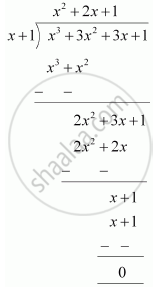Find the remainder when x^3 + 3x^2 + 3x + 1 is divided by x+1. - Mathematics

Find the remainder when x3 + 3x2 + 3x + 1 is divided by x+1.

Solution 1

Let p(x) = x3 + 3x2 + 3x + 1

x+1 = 0 ⇒ x = -1

∴ Remainder = p(-1) = (-1)3 + 3(-1)2 + 3(-1) + 1

= -1 + 3 - 3 + 1

= 0

Therefore, the remainder is 0.

Solution 2

By long division,Therefore, the remainder is 0.

Concept: Remainder Theorem
Is there an error in this question or solution?

APPEARS IN

NCERT Class 9 Maths
Chapter 2 Polynomials
Exercise 2.3 | Q 1.1 | Page 40